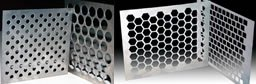## Perforated Metal Styles## Processing Machines# Calculation of Sheet Metal Weight

Calculation of perforated metal sheet weight per square meter can be done as reference below:

ps = absolute (specific) weight (Kg) , v/p = open area (%) , s = thickness mm , kg = [s*ps*(100-v/p)]/100

Open area calculation when holes 60Â° staggered:
V/p = open area (%) ,D = holes diameter (mm) ,P = holes pitch (mm) ,v/p = (D2*90,7)/p2

S = Thickness in mm   D = Wire Diameter in mm     P = Pitch in mm    V = Open Area %

Some patterns as following: